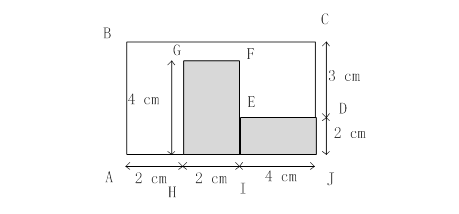### Sample Problem

The perimeter of the un-shaded part of the figure below is cm.#### Solution

The perimeter is the sum of AB, BC, CD, DE, EF, FG, GH and HA. So it is (3 + 2) + (2 + 2 + 4) + 3 + 4 + (4 - 2) + 2 + 4 + 2 = 30 cm.